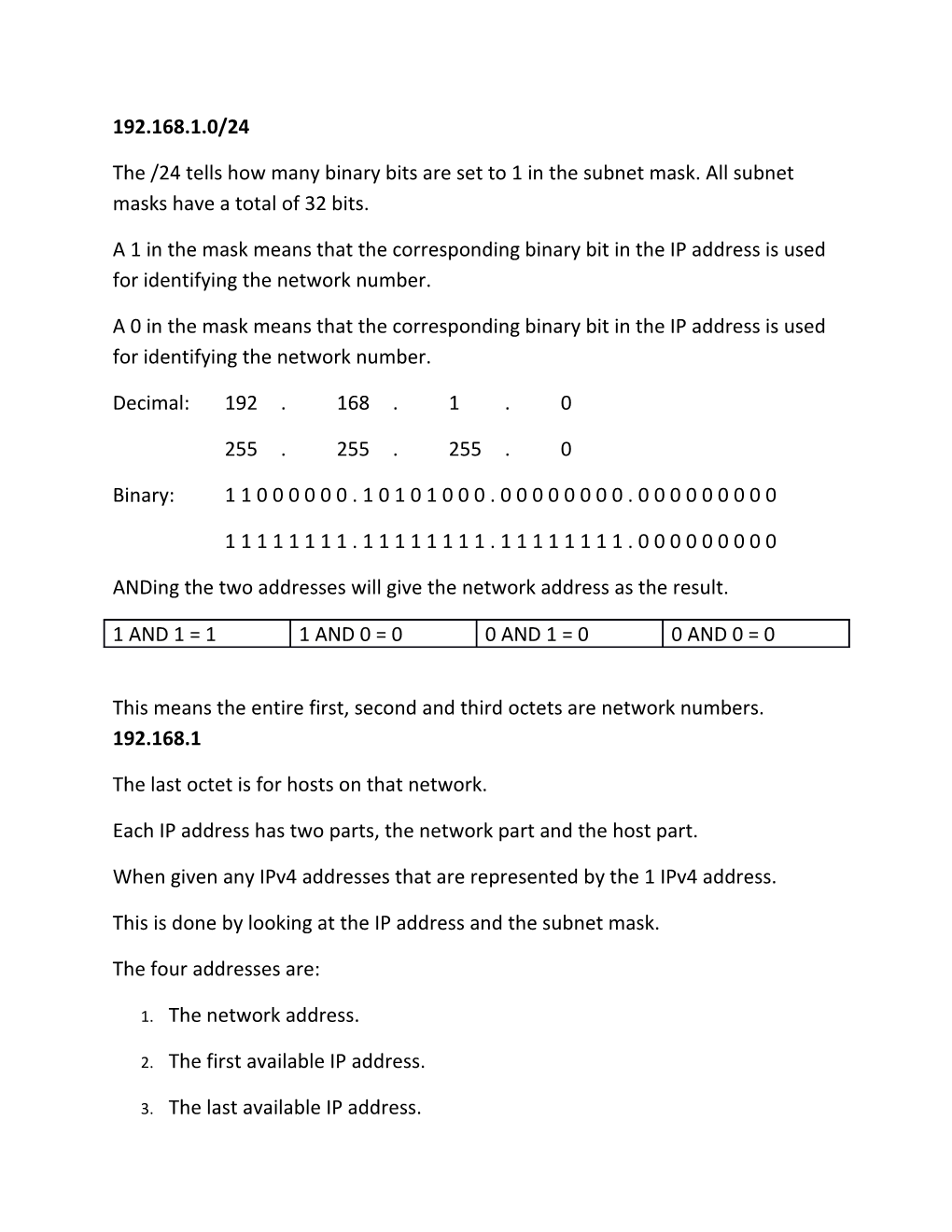# Anding the Two Addresses Will Give the Network Address As the Result192.168.1.0/24

The /24 tells how many binary bits are set to 1 in the subnet mask. All subnet masks have a total of 32 bits.

A 1 in the mask means that the corresponding binary bit in the IP address is used for identifying the network number.

A 0 in the mask means that the corresponding binary bit in the IP address is used for identifying the network number.

Decimal:192.168.1.0

255.255.255.0

Binary:1 1 0 0 0 0 0 0 . 1 0 1 0 1 0 0 0 . 0 0 0 0 0 0 0 0 . 0 0 0 0 0 0 0 0 0

1 1 1 1 1 1 1 1 . 1 1 1 1 1 1 1 1 . 1 1 1 1 1 1 1 1 . 0 0 0 0 0 0 0 0 0

ANDing the two addresses will give the network address as the result.

1 AND 1 = 1 / 1 AND 0 = 0 / 0 AND 1 = 0 / 0 AND 0 = 0

This means the entire first, second and third octets are network numbers. 192.168.1

The last octet is for hosts on that network.

Each IP address has two parts, the network part and the host part.

When given any IPv4 addresses that are represented by the 1 IPv4 address.

This is done by looking at the IP address and the subnet mask.

2. The first available IP address.
3. The last available IP address.

• Found by setting all binary bits in the host portion to 0.

Given 192.168.1.10/24/24 = 255.255.255.0

The network portion is the first 3 octets.

192.168.1

The host portion is the last octet.

.10

Set the host portion to all zeros to find the network address.

Binary

192.168.10.00000000

Decimal

The first available IP address for the network is found by setting all binary bits in the subnet mask to zero, except the right most bit.

Given 192.168.1.10/24

The network portion is the first 3 octets.

192.168.1

The host portion is the last octet.

.10

Set the host portion to all zeros except the right most bit.

Binary

192.168.10.00000001

Decimal

192.168.10.1is the first available IP address.

Given 192.168.10.10/25

The subnet mask has 25 bits turned on, which is a 1. The mask has a total of 32 bits in it. Therefore, 32-25 = 7 host bits

Network bits  Host bits = 32

Binary 1 Binary 0

Number of hosts on a network is calculated by 2n – 2.

Where n is the number of host bits for 192.168.10.10/25

25 network bits, therefore 7 host bits (25 + 7 = 32)

n = 7

27 -2 = 128 – 2 = 126

The minus 2 comes from subtracting.

• 1 for the network address

The last available IP address is found by setting all binary bits in the host portion to 1, except the right most bit.

Given 192.168.1.10/24

The network portion is the first 3 octets.

192.168.1

The host portion is the last octet.

.10

Set the host portion to all ones, except the right most bit

Binary

192.168.10.11111110

Decimal

192.168.1.254 is the last available IP address.

The broadcast address found by setting all binary bits in the host portion to 1.

Given 192.168.1.10/24

The network portion is the first 3 octets.

192.168.1

The host portion is the last octet.

.10

Set the host portion to all ones.

Binary

192.168.10.11111111

Decimal

Given 192.168.10.10/25

The address is a class C address, which has a default mask of /24, which is 255.255.255.0

The given mask is /25, which means that 1 bit has been changed from a host representing bit to a network representing bit.

/24 = 192.168.10.0

11111111 .11111111 .11111111 . 00000000

24 network bits 7 host bits

/25 =

1111111 .11111111 .11111111 . 10000000

25 network bits 7 host bits

Once the bit has become a network bit, you now “own” the bit. The fact that you own it means you control it and the values it can represent.

The original network with a /24 is one network and with 8 bits for hosts.

2n - 2 = 254

The new mask has an extra network bit, so you can now have control over one bit. This makes 2 new sub-networks because that bit can be on or off.

2n = networks.

n = bits borrowed.

/24  /25 is 1 bit borrowed.

21 = 2 networks.

192.168.10.0/25

11111111 .11111111 .11111111 . 10000000

Octet all network One more 7 host bits

network bit

First sub-network is found by turning the bit you now own to off

192 .168 .10 .0 0000000

Your bit 7 host bits all set to zero for the network address

= 192.168.10.0

192 .168 .10 .0 0000001

=0 = 1

0 + 1 = .1

192 .168 .10 .0 1111110

=0 = 126

0 + 126 = .126

192 .168 .10 .0 1111111

=0 = 127

0 + 127 = .127

Now turn on the bit you own

192 .168 .10 .1 0000000

= 128 = 0

128 + 0 = .128

192 .168 .10 .1 0000001

= 128 = 1

128 + 1 = .129

192 .168 .10 .1 1111110

= 128 = 126

128 + 126 = .254